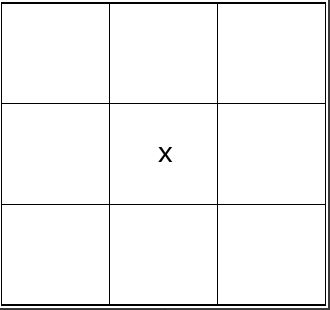# Magic Square Problem

Logic Level 2

What is the value of $x$ in the order 3 magic square if the magic constant is 19?Definition: the magic constant of a magic square has the value of the sum of all rows, diagonals, and columns of the square.

×